# 旷视CV Master计算摄影学训练营课程笔记1

## Aug 9,2021   8064 words   29 min

#### 0.课程简单介绍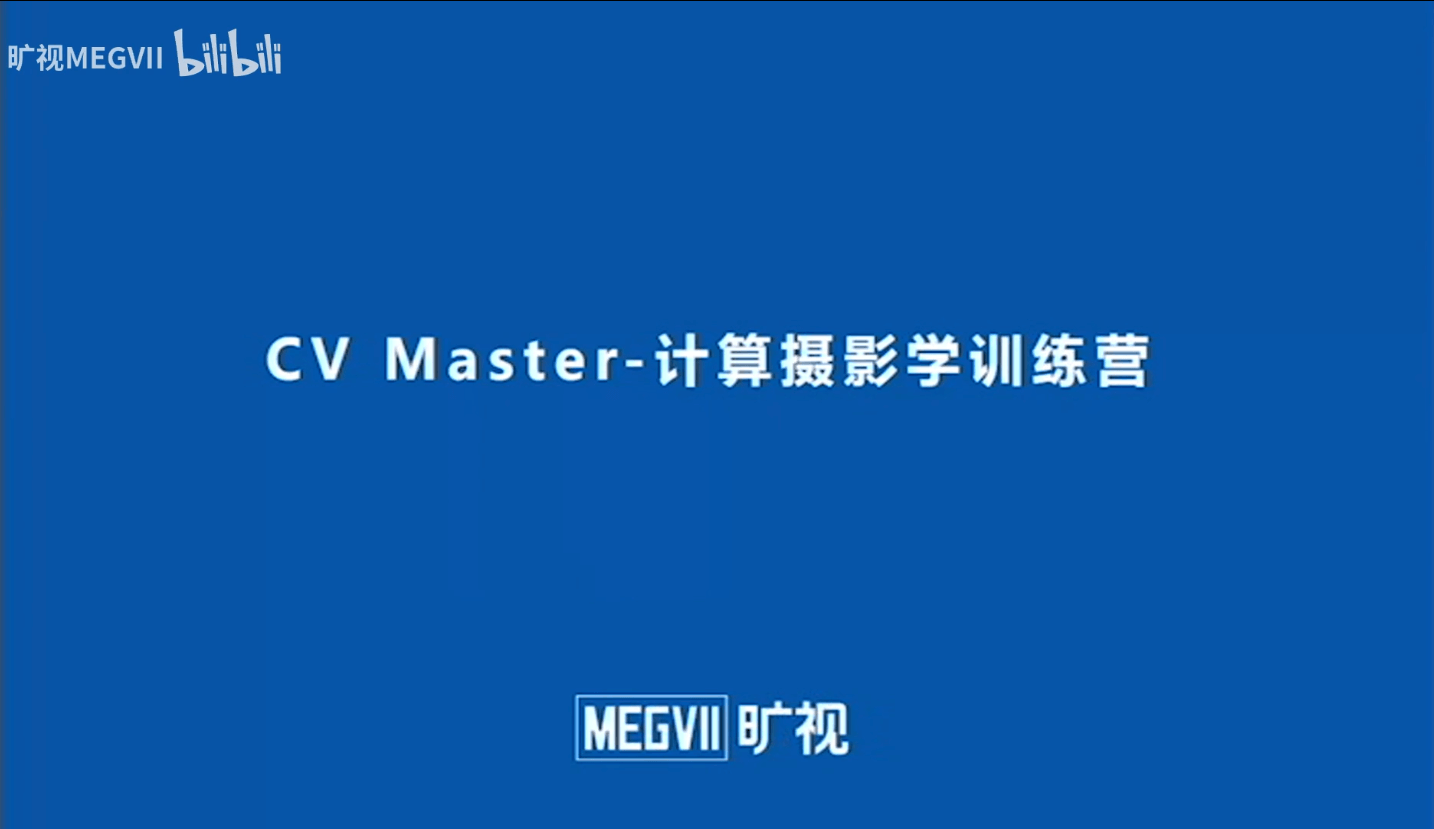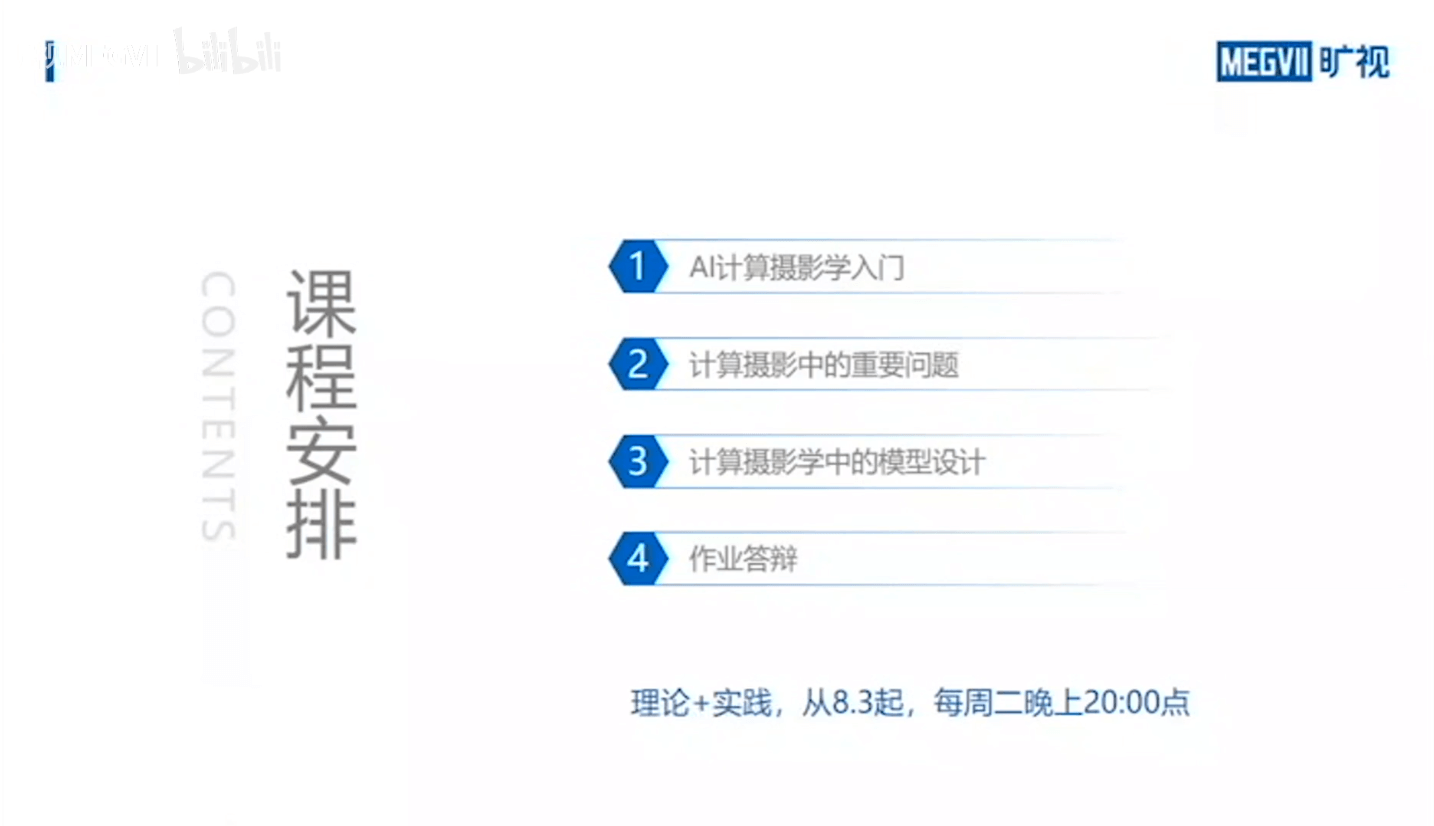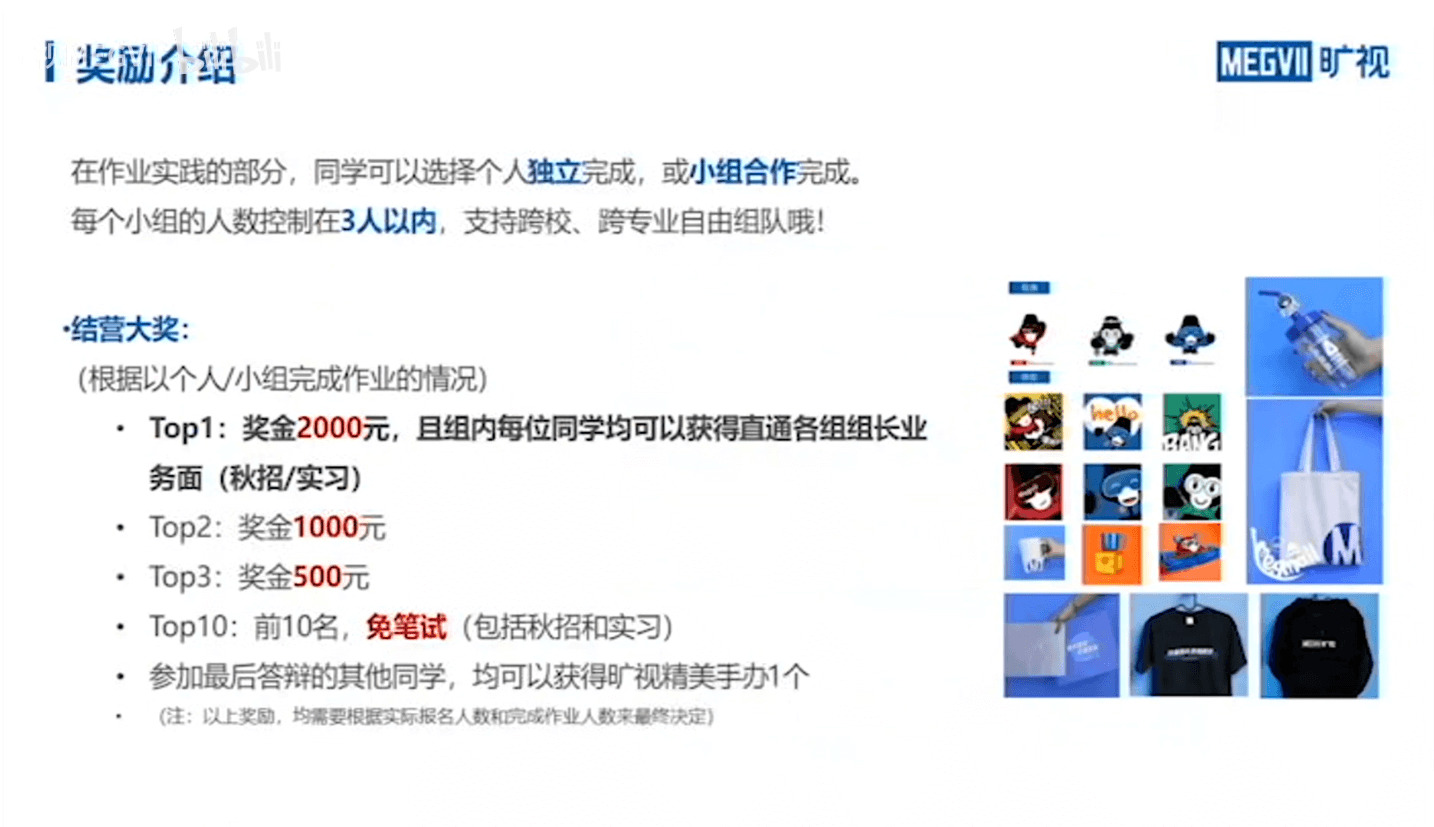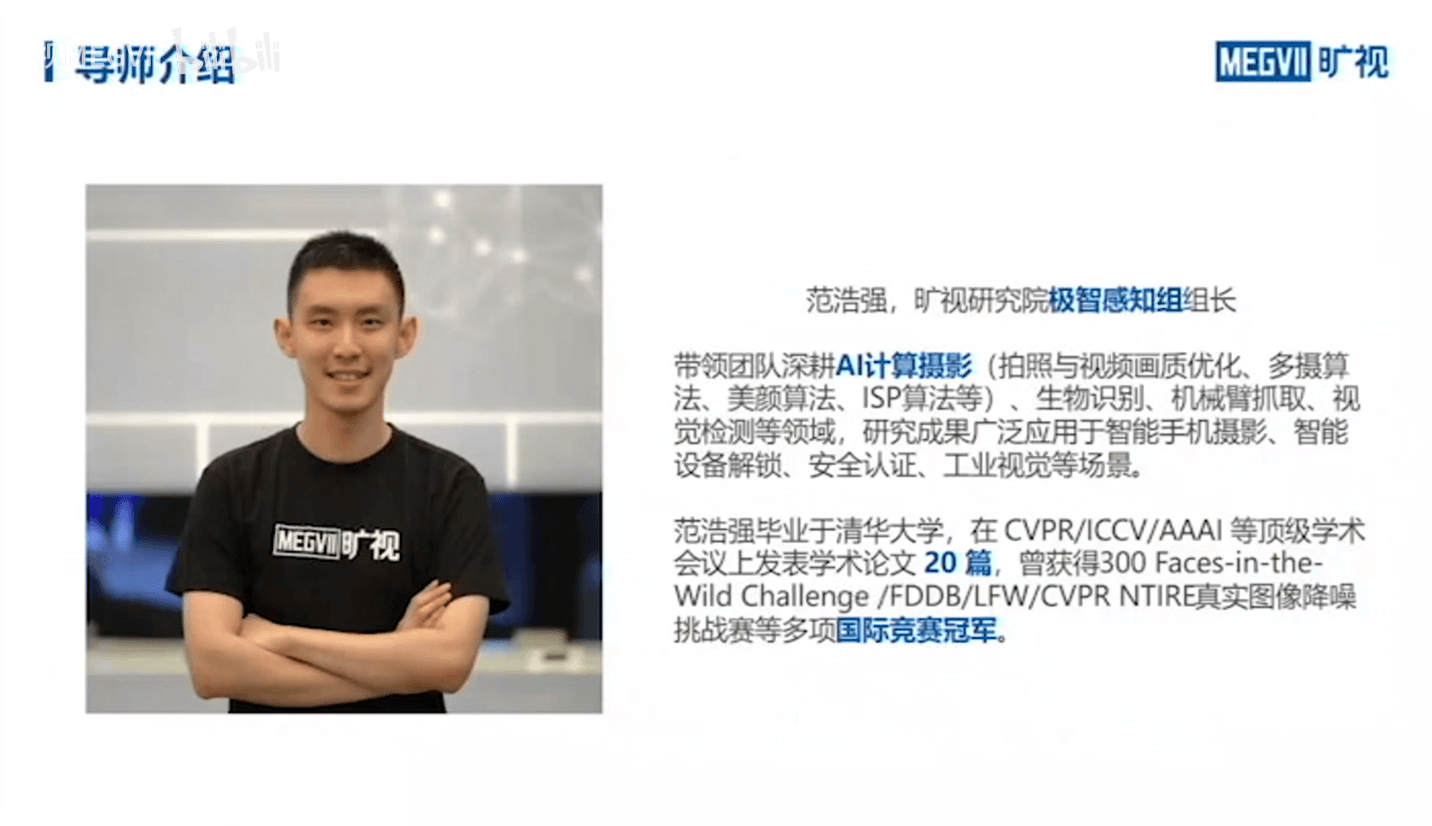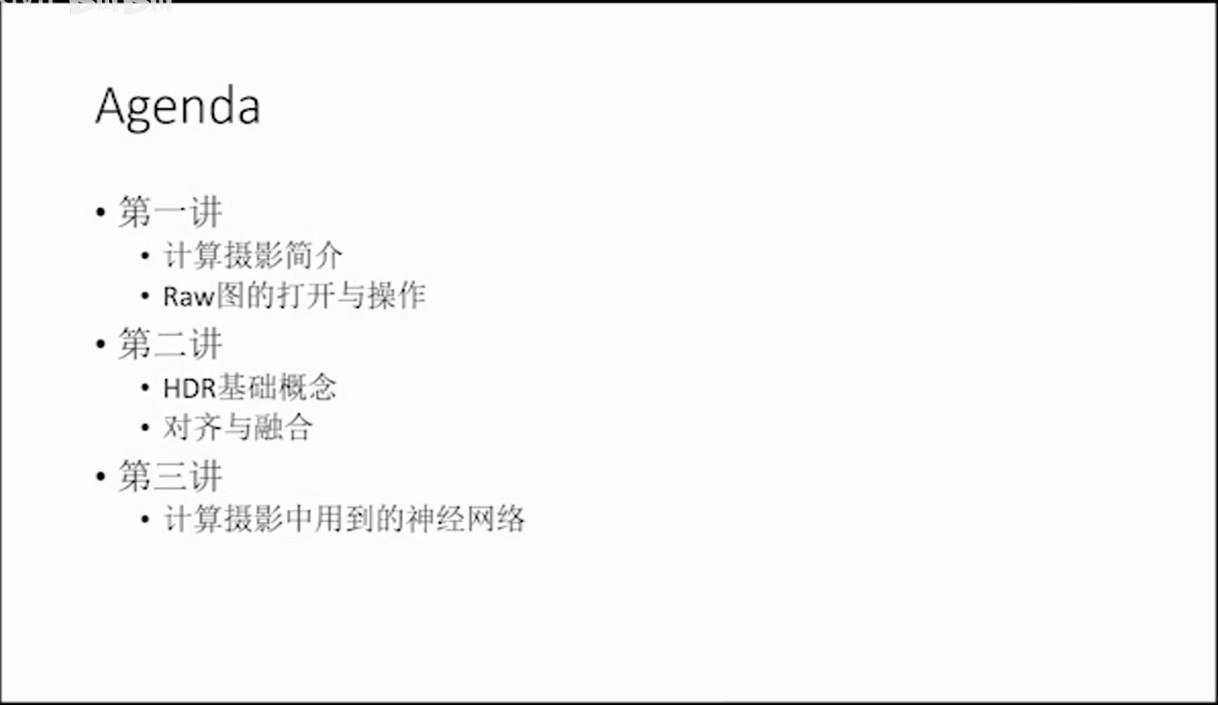#### 2.相机的Raw图

##### (2) Raw图的读取

``````from matplotlib import pyplot as plt  # 可视化相关
import rawpy  # Raw图解析相关
import numpy as np  # 矩阵运算相关

# 读取并解析Raw图
array_data = img.raw_image_visible

# 遍历像素统计灰度直方图
bins =  * 1024
for i in range(array_data.shape):
print(i + 1, '/', array_data.shape)
for j in range(array_data.shape):
bins[array_data[i, j]] += 1

# 输出相关信息检查灰度统计是否正确
print('Image width:', array_data.shape)
print('Image height:', array_data.shape)
print('Total pixel:', array_data.shape * array_data.shape)
print('Total pixel in practice:', np.sum(bins))
print('Datatype:', array_data.dtype)

# 绘制灰度直方图
plt.figure(1)
plt.bar(range(len(bins)), bins)

# 绘制Raw图
plt.figure(2)
plt.imshow(array_data, cmap='gray')

# 可视化
plt.show()
``````

##### (3) Raw图的显影

Raw图的显影可以理解为是将Raw图的数据转换为普通8bit图像的过程。需要注意的是，这里的显影和刚刚的Raw图可视化是不同的。可视化只是将原始数据不经过任何处理的显示出来，而显影则是一种“转换”过程。最直观的区别就是量化级数不同，raw图一般是10bit，普通jpg是8bit，需要转换。另外不同之处在于raw图是全色影像，需要经过计算才能变成多波段彩色影像。此外，还要考虑底噪的影响。具体来说，还需要经过下图所示的步骤。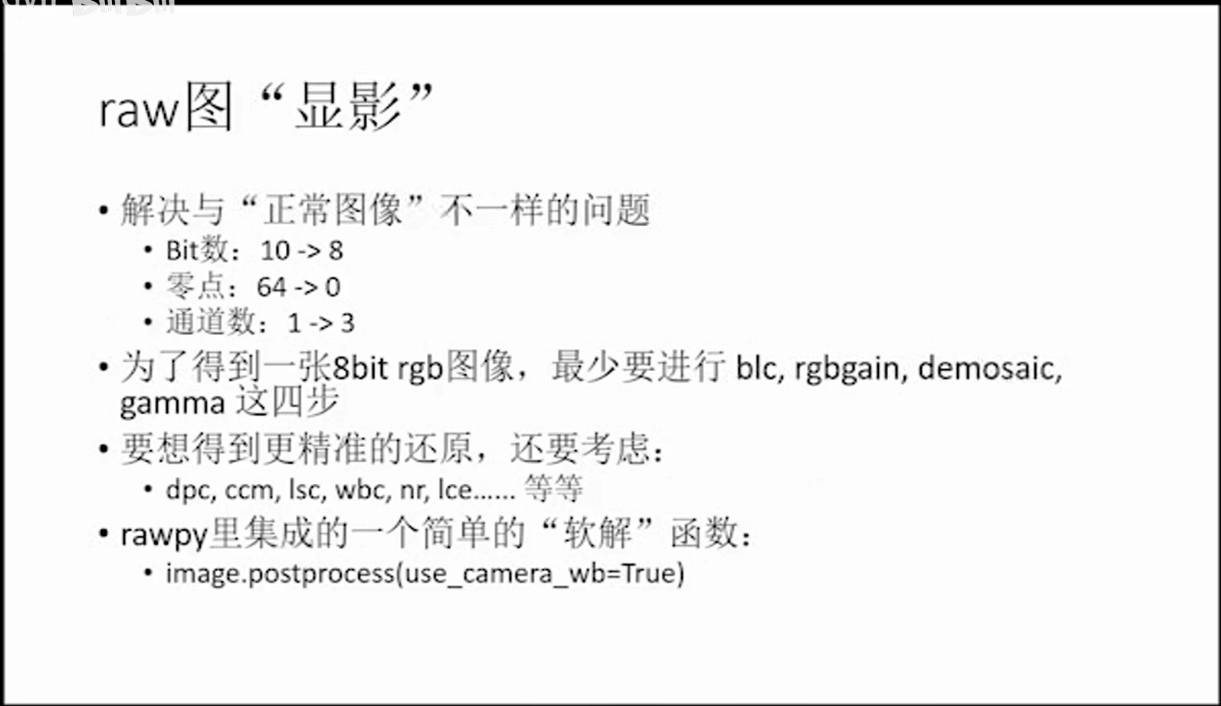至少要经过blc、rgbgain、demosaic、gamma最基本的这四步，才可以得到一个8bit彩色影像。如果要更好的效果，当然需要考虑更多因素。在Rawpy中，提供了一个简单的“软解”函数postprocess()，可以对Raw数据进行解析。

``````from matplotlib import pyplot as plt  # 可视化相关
import rawpy  # Raw图解析相关

# 读取并解析Raw图
array_data = img.raw_image_visible

# 利用Rawpy进行软解
pp_data = img.postprocess(use_camera_wb=True)

# 输出相关信息
print("Raw datatype:", array_data.dtype)
print("Postprocess datatype:", pp_data.dtype)

# 可视化
plt.figure(1)
plt.imshow(array_data, cmap='gray')
plt.figure(2)
plt.imshow(pp_data, cmap='gray')
plt.show()
``````

``````from matplotlib import pyplot as plt  # 可视化相关
import rawpy  # Raw图解析相关
import numpy as np  # 矩阵运算相关
import cv2  # 影像读取相关

# 读取Raw图

# 直接解析
out_pp = img.postprocess(use_camera_wb=True)

# 先进行处理再解析
pixels = img.raw_image_visible.astype('float32')
xs, ys = np.mgrid[:pixels.shape, :pixels.shape]
xs = np.float32(xs) - xs.mean()
ys = np.float32(ys) - ys.mean()
rs = (xs ** 2 + ys ** 2) ** 0.5 / xs.shape
shading = 1 + rs ** 2 * 3
# black level
bl = 64
pixels = bl + (pixels - bl) * 3 * shading
img.raw_image_visible[:] = pixels.clip(0, 1023)

# 利用Rawpy进行软解
out = img.postprocess(use_camera_wb=True)

# 读取手机直出图像作为对比
img_phone_rgb = cv2.cvtColor(img_phone, cv2.COLOR_BGR2RGB)

# 可视化
plt.figure(1)
plt.title("postprocess")
plt.imshow(out_pp, cmap='gray')
plt.figure(2)
plt.title("postprocess with lsc")
plt.imshow(out, cmap='gray')
plt.figure(3)
plt.title("phone")
plt.imshow(img_phone_rgb, cmap='gray')
plt.show()
``````

#### 3.手动进行postprocess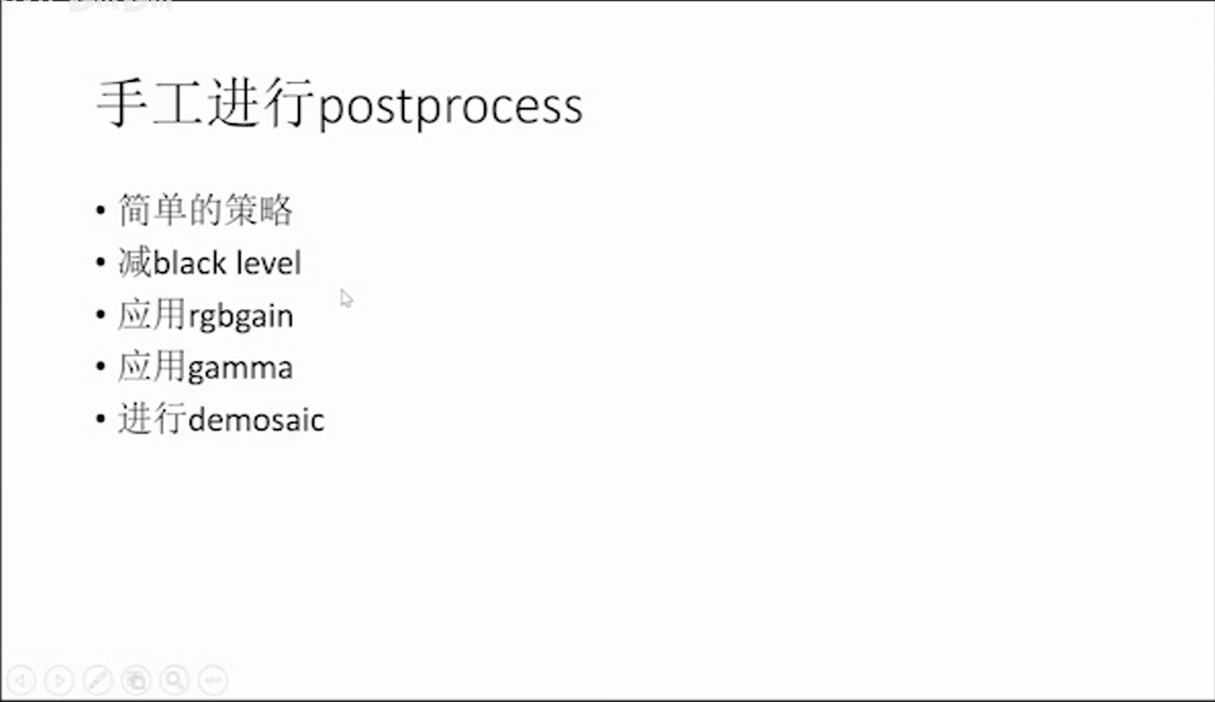正如前面说的，软解最基本可以分为减black level、应用rgbgain、应用gamma、进行demosaic。下面简单分别进行介绍一下。

##### (1) 减black level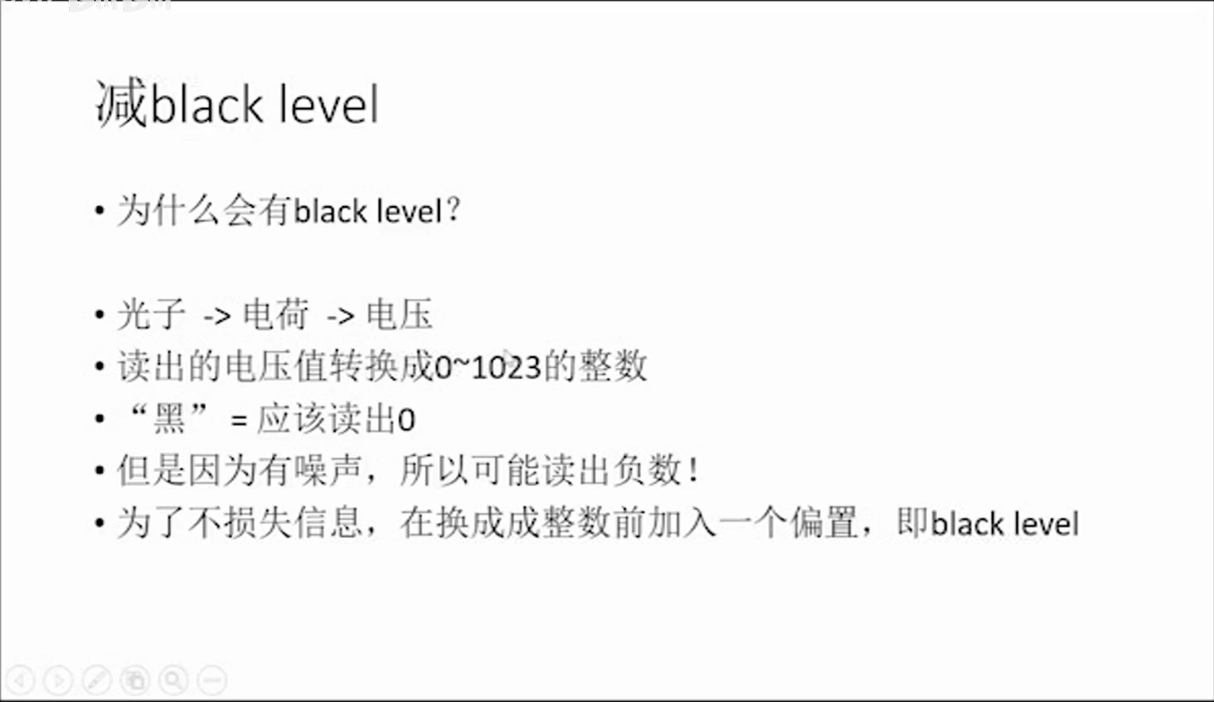为什么黑色不是0？一方面当然是由于各种噪声，导致读出来的值存在干扰。另一方面这其实是在设计的时候故意而为之的。如果不加一个偏置，由于传感器热噪声等很多因素，很有可能读出来负数。但是保存的数据类型为uint类型，所以负数也会保存为0。这样显而易见会丢失信息。

``````from matplotlib import pyplot as plt  # 可视化相关
import rawpy  # Raw图解析相关
import numpy as np

# 读取Raw图
array_data = img.raw_image_visible

# 遍历像素统计灰度直方图
bins =  * 1024
for i in range(array_data.shape):
print(i + 1, '/', array_data.shape)
for j in range(array_data.shape):
bins[array_data[i, j]] += 1

for i in range(55, 75):
print(i, ":", bins[i])

mean_value = np.mean(array_data)
print("Mean value:", mean_value)

# 绘制图像
plt.figure(1)
plt.bar(range(55, 75), bins[55:75])
plt.figure(2)
plt.title("array_data")
plt.imshow(array_data)
plt.show()
``````

##### (2) 应用rgbgain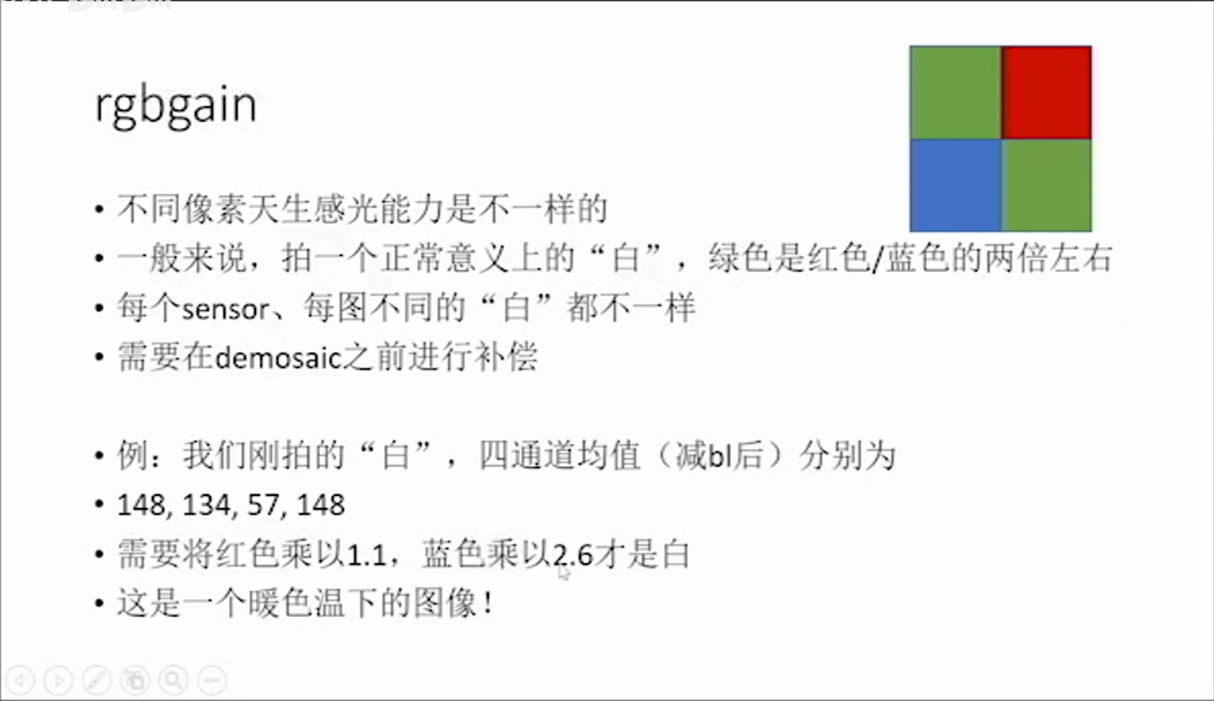如上图所示，由于在传感器表面加了彩色绿光片，而且再加上Bayer Patter，导致了不同像素天生感光能力是不一样的。因此，在进行进一步处理之前，还需要对RGB色彩进行补偿。

##### (4) 进行demosaic

Demosaic简单来说就是根据Bayer Pattern获取到的R、G、B像素值，通过算法生成每个像素的RGB值，从而形成三通道RGB影像。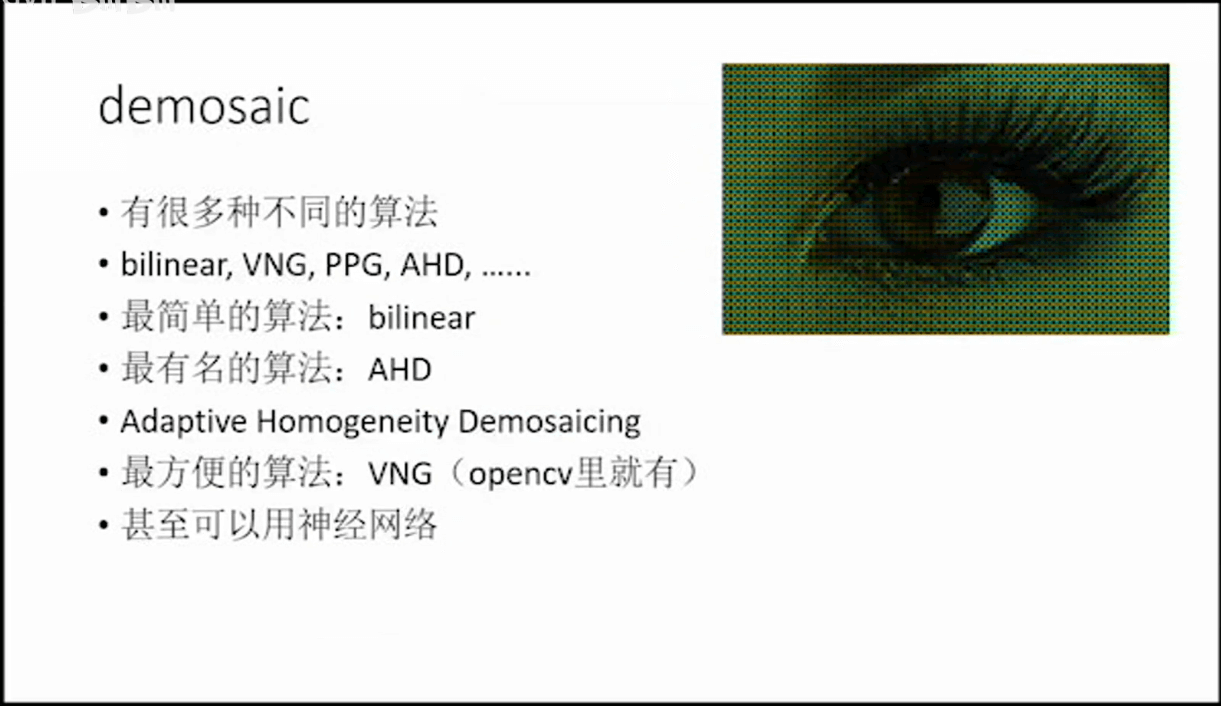一个简单的双线性Demosaic算法示意如下。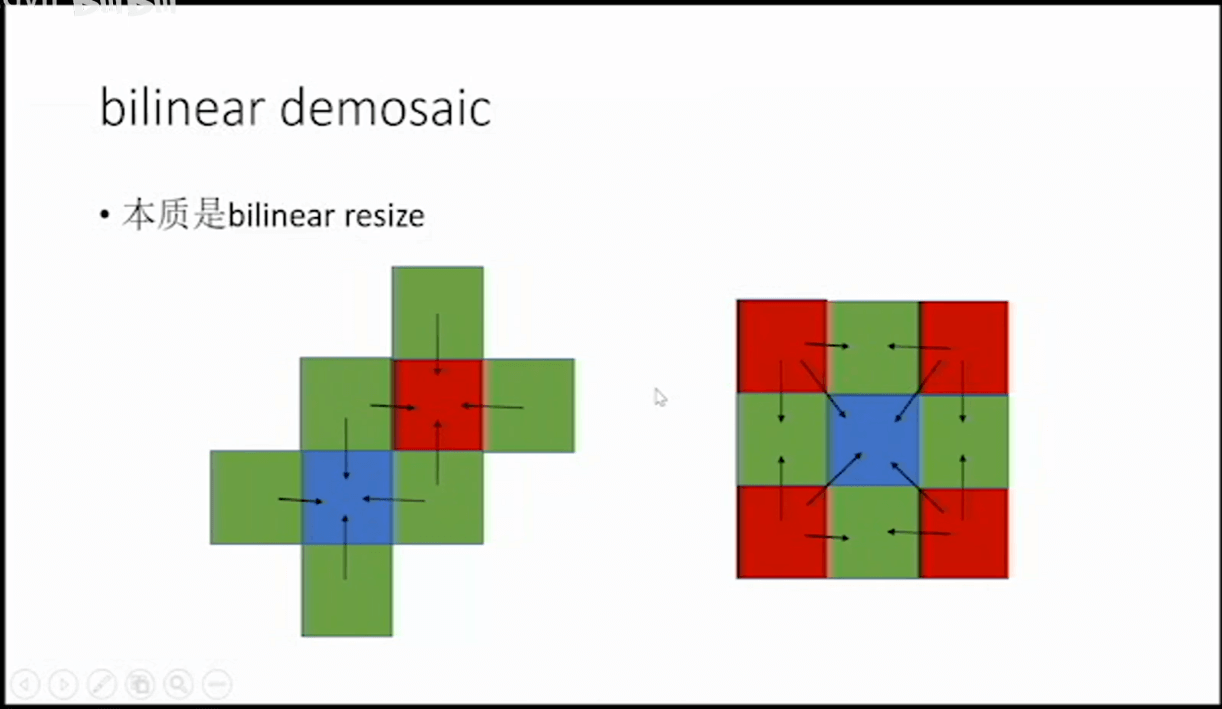首先如左图所示，每个红色和蓝色的上、下、左、右周围都有四个绿色，所以很简单的，我们取这四个绿色的平均值，将其作为绿色赋给对应的红色或者蓝色。这样原本红色的像素就有红色和绿色，原本蓝色的像素就有蓝色和绿色，原本绿色的现在还是只有绿色。或者换句话说，影像中所有像素的绿色就都补齐了。然后我们可以考虑填补红色，如右图所示。对于上面中间的绿色像素，我们就找左右两边的红色像素，然后取平均，作为该绿色像素的红色分量。同理，第二行左边的绿色像素也找离它最近的两个红色(上下两个)，然后取平均。而对于蓝色像素，则寻找四个角的红色像素，然后取个平均作为该蓝色像素的红色分量。经过这样的操作，影像中所有像素的红色分量就都被补齐了。最后，我们可以类似地补全蓝色分量，就可以把每个像素的RGB分量都补全了。

#### 4.课程作业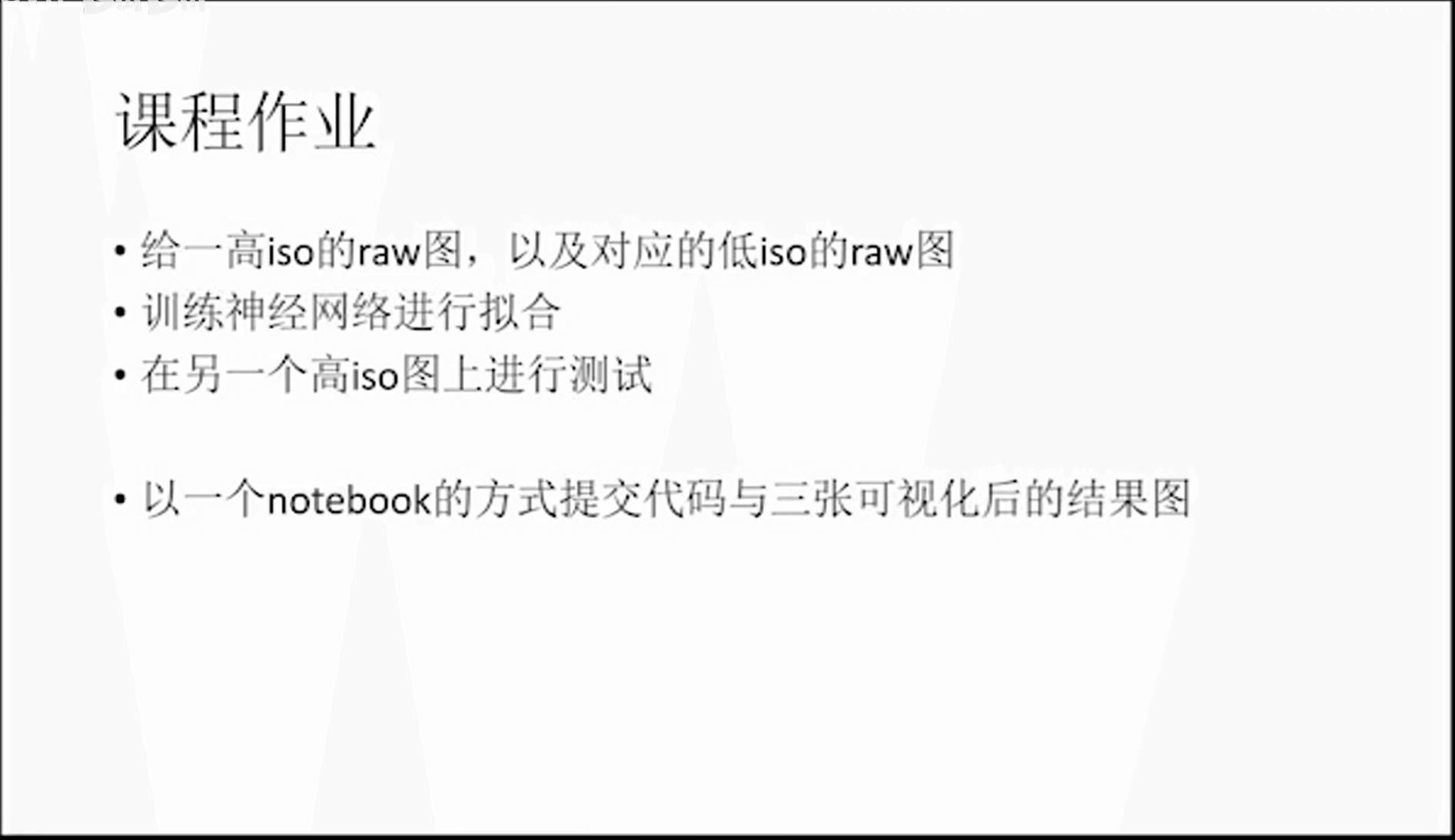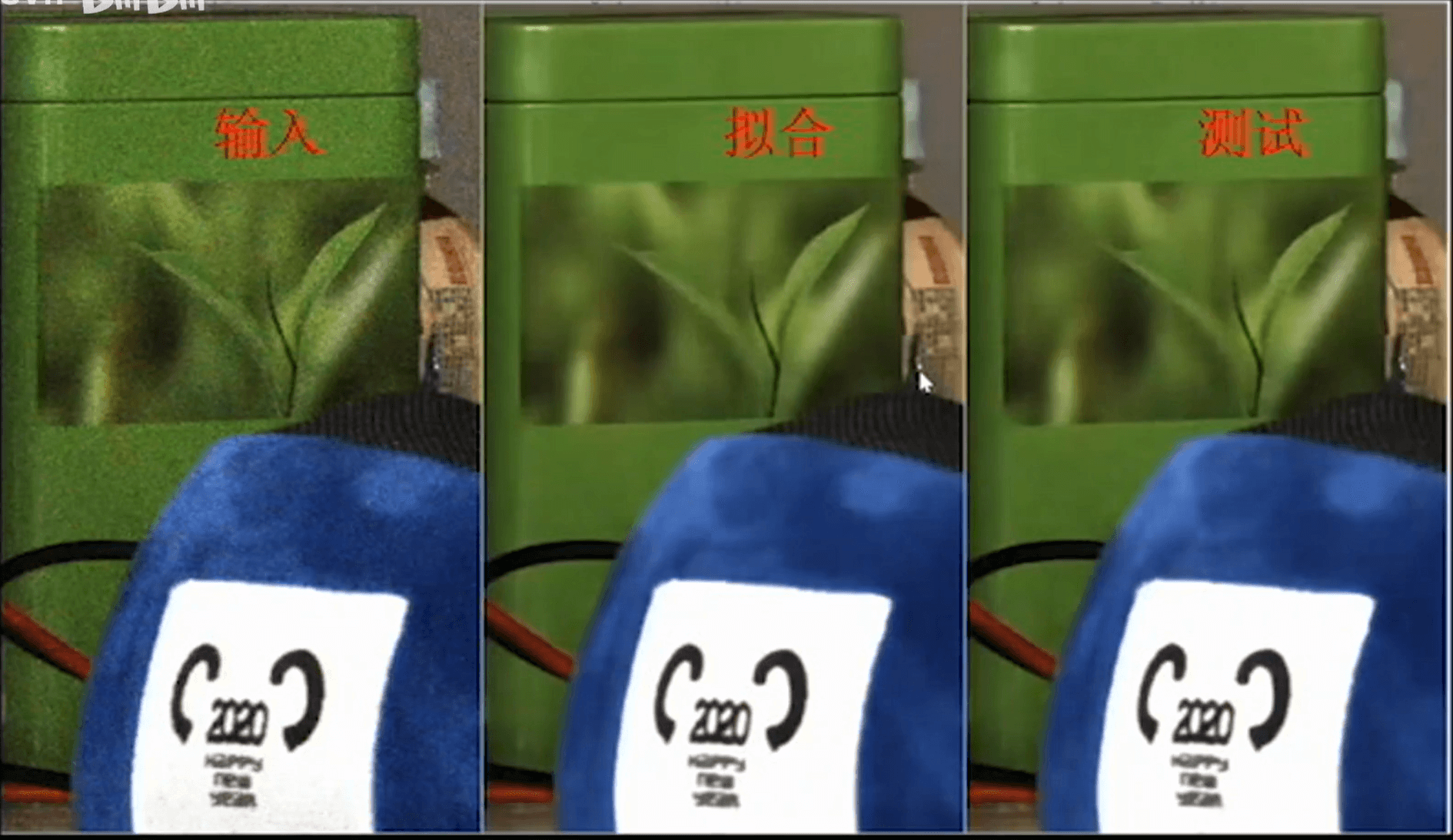#### 5.参考资料

•  https://pypi.org/project/rawpy/
•  https://github.com/letmaik/rawpy-notebooks
•  https://www.zhihu.com/question/27467127
•  https://github.com/zhaoxuhui/RawImageProcessingDemo